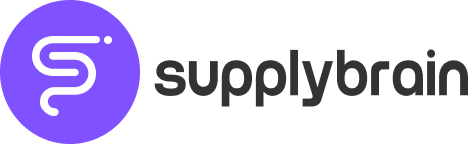## What is Economic Order Quantity?

Economic Order Quantity is basically the ideal quantity you must order when trying to minimize inventory costs. These costs include holding inventory, shortage and costs involving lead time, such as transportation and logistics.

The model is used at the continuous review inventory policy, in which the level of inventory is always monitored, and the order is made at specific times. EOQ assumes a constant demand and a fixed deplete rate.

In short terms, the EOQ calculate the proper reorder point and quantity, to make sure an optimal replenishment with no shortages. EOQ was developed in 1913 by Ford W. Harris and is used until now.

## The purpose of Economic Order Quantity

The EOQ is an optimization model that will try to get in the most economic scenario of order. Trying to equalize holding stock and order. So, it’s a model of optimization for order and production costs.

It is a model that will help you set how much you should order and how many times. It usually considers a long cycle, such as the annual revenue of a company.

The reason to apply optimization models is to arrive at the best possible scenario. EOQ seeks to find the optimal order costs. At the same time, you may economize buying more items, you should for instance consider the holding costs.

You can face, for example, a risk of not being able to sell all of these items. Or the risk of loosing items for obsolescence. A series of costs related to maintain items and making order.

Therefore, what EOQ does is finding the balance between those points, cutting costs, and increasing profit in each order. So, it will look at the annual demand to find those optimized values.

## Economic Order Quantity Formula

As we established, EOQ is calculated by minimizing total cost per order. If we think in the first order as derivative of zero, other components are costs of holding inventory and ordering.

So, let’s pass for each element that composes the EOQ formula before explaining more about the concept:

D: Annual Quantity Demanded

Q: Volume per Order

S: Ordering Cost

C: Unit Cost

H: Holding Cost

I: Carrying Cost

K: Transaction Cost

At the end we have a basic formula for EOQ:

Q = √2DS/H

## EOQ’s graphic representation

When trying to answer the question about the assumption of costs estimations, the specialist in supply chain Nicolas Vandeput proposes two ways: the first one is a graphic representation of supply chain costs and the second a mathematical model.

The graph would show that when you are close to the optimal order quantity, the total cost curve is rather flat. So, if you stay close to Q, you’ll get a total cost close to the optimal:

## EOQ trough time

Although the first EOQ model was developed in 1913, a lot of extensions were proposed afterwards. It’s a model that’s been thoroughly discussed in the academic literature and because of that is a very solid model.

One of the extensions is the Production Rate will adapt EOQ for a internal production process, instead of considering an external supplier. For this model this model, Vandeput concludes that producing too much and too fast doesn’t help reducing overall costs.

Another extension is the Backorders. It proposes that allowing some backorders are actually optimal for supply chains, instead of aiming for 100% service level. In other words, having backorders are good are better than serving every order from on-hand inventory.

The extension of discounts incorporates the discounts offered by suppliers. The good thing about this extension is that it will optimize considering lower prices from larger orders.

## Conclusion

The EOQ model is a good model for small business with a large and variable inventory. It is one of many ways to a company make itself more efficient and profitable.

A bad side of EOQ is that it assumes that the demand will be the same over time. Also, the calculation assumes that ordering prices and costs remain constant.

With an artificial intelligence tool, the definition of the Economic Order Quantity aligned to the stock policy can be more assertive. With Supply Brain we apply a daily review methodology and optimize the SLA. Thus, we avoid excess and rupture of stock, taking into consideration freight costs and costs to keep a product in stock. Want to know our tool? Get in touch with us!### Thiago Gomes

[email protected]

##### Belo Horizonte

Rua Carlos Alves, 88 -101 – São José

##### São Paulo

Cubo Itaú – Alameda Vicente Pinzon, 54 – Vila Olímpia